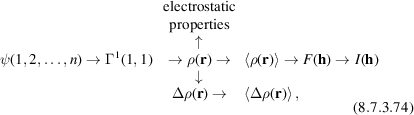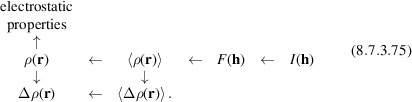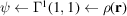International
Tables for
Crystallography
Volume C
Mathematical, physical and chemical tables
Edited by E. Prince

International Tables for Crystallography (2006). Vol. C, ch. 8.7, pp. 721-722

## Section 8.7.3.5. Quantitative comparison with theory

P. Coppens,a Z. Sub and P. J. Beckerc

a732 NSM Building, Department of Chemistry, State University of New York at Buffalo, Buffalo, NY 14260-3000, USA,bDigital Equipment Co., 129 Parker Street, PKO1/C22, Maynard, MA 01754-2122, USA, and cEcole Centrale Paris, Centre de Recherche, Grand Voie des Vignes, F-92295 Châtenay Malabry CEDEX, France

#### 8.7.3.5. Quantitative comparison with theory

| top | pdf |

Frequently, the purpose of a charge density analysis is comparison with theory at various levels of sophistication. Though the charge density is a detailed function, the features of which can be compared at several points of interest in space, it is by no means the only level at which comparison can be made. The following sequence represents a progression of functions that are increasingly related to the experimental measurement.where the angle brackets refer to the thermally averaged functions.

The experimental information may be reduced in the opposite sequence:The crucial step in each sequence is the thermal averaging (top sequence) or the deconvolution of thermal motion (bottom sequence), which in principle requires detailed knowledge of both the internal (molecular) and the external (lattice) modes of the crystal. Even within the generally accepted Born–Oppenheimer approximation, this is a formidable task, which can be simplified for molecular crystals by the assumption that the thermal smearing is dominated by the larger-amplitude external modes. The procedure (8.7.3.65)requires an adequate thermal-motion model in the structure-factor formalism applied to the experimental structure amplitudes. The commonly used models may include anharmonicity, as described in Volume B, Chapter 1.2(ITB, 2001), but assume that a density function centred on an atom can be assigned the thermal motion of that atom, which may be a poor approximation for the more diffuse functions.

The missing link in scheme (8.7.3.75)is the sequence. In order to describe the wavefunction analytically, a basis set is required. The number of coefficients in the wavefunction is minimized by the use of a minimal basis for the molecular orbitals, but calculations in general lead to poor-quality electron densities.

If the additional approximation is made that the wavefunction is a single Slater determinant, the idempotency condition can be used in the derivation of the wavefunction from the electron density. A simplified two-valence-electron two-orbital system has been treated in this manner (Massa, Goldberg, Frishberg, Boehme & La Placa, 1985), and further developments may be expected.

A special case occurs if the overlap between the orbitals on an atom and its neighbours is very small. In this case, a direct relation can be derived between the populations of a minimal basis set of valence orbitals and the multipole coefficients, as described in the following sections.

### References

International Tables for Crystallography (2001). Vol. B, edited by U. Shmueli. Dordrecht: Kluwer Academic Publishers.Google Scholar
Massa, L., Goldberg, M., Frishberg, C., Boehme, R. F. & La Placa, S. J. (1985). Wave functions derived by quantum modeling of the electron density from coherent X-ray diffraction: beryllium metal. Phys. Rev. Lett. 55, 622–625.Google Scholar